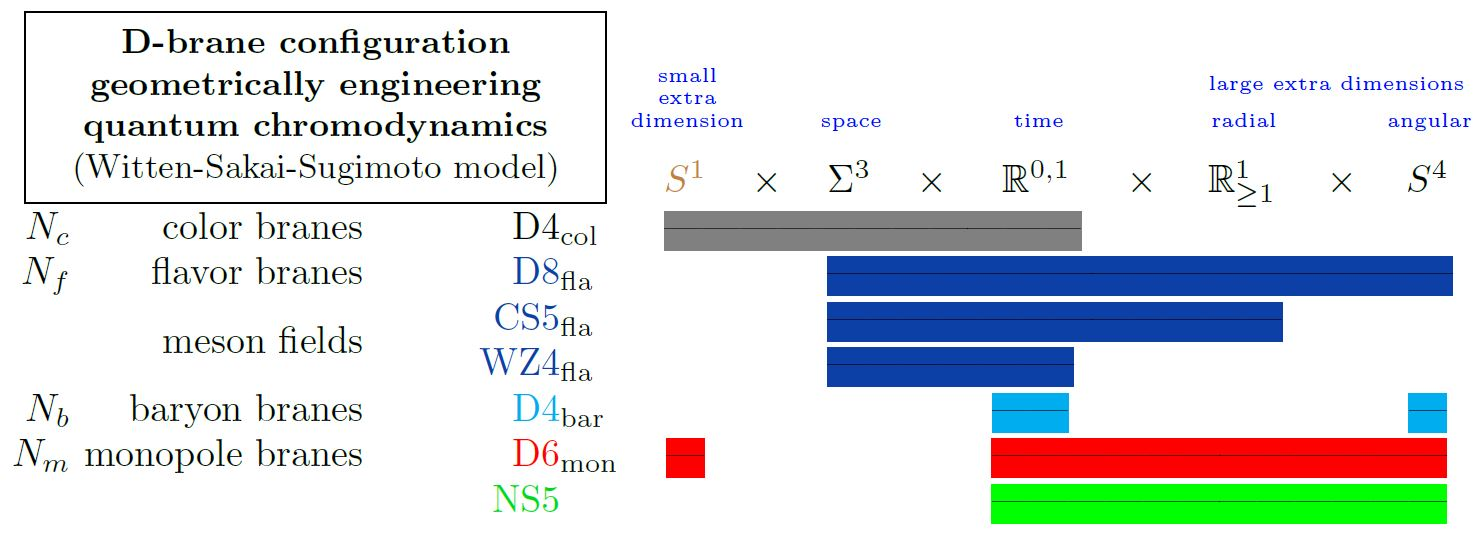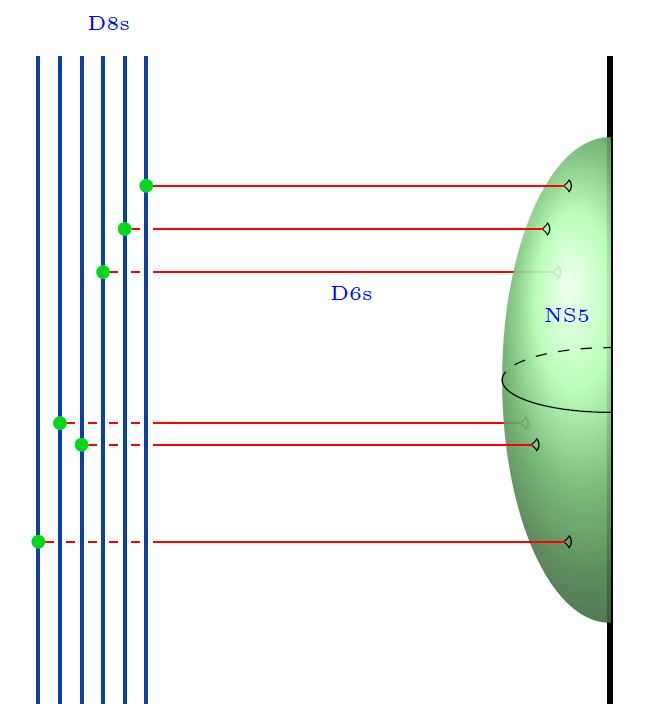Contents

# Contents

## Idea

The D-brane in type II string theory of dimension $8+1$.

## Properties

### As black branes in massive IIA supergravity

As black brane-solutions, D8-branes appear in massive type IIA supergravity (“Romans supergravity”)/massive type IIA string theory

Since the normal n-sphere around a D8-brane (with its 9-dimensional worldvolume) in 10-dimensional spacetime is a 0-sphere, D8-brane charge is measured by the RR-field 0-flux form $F_0$. This behaves like a cosmological constant in the corresponding D=10 supergravity (“Romans supergravity”). Its presences changes ordinary type IIA string theory to what is called massive type IIA string theory, whose relation to the web of dualities in string theory remains not well understood. In particular the duality between type IIA string theory and M-theory remains ill-understood for massive type IIA string theory.

### Bound states

For the bound states and brane intersections involving D8-branes, see at

### As geometric engineering of flavour physics

In geometric engineering of flavor physics in intersecting D-brane models, the flavour degrees of freedom come from open strings ending on spacetime-filling D-branes (Karch-Katz 02).

Specifically in the Sakai-Sugimoto model geometrically engineering something close to actual quantum chromodynamics (Sakai-Sugimoto 04, Sakai-Sugimoto 05), flavour is encoded in the presence of D8-branes in the model:Here we are showing

1. the color D4-branes;

2. the flavor D8-branes;

with

1. the 5d Chern-Simons theory on their worldvolume

2. the corresponding 4d WZW model on the boundary

both exhibiting the meson fields;

3. (see at WSS-model – Baryons);

4. (see at D6-D8-brane bound state);

5. the NS5-branes (often not considered here).

Table of branes appearing in supergravity/string theory (for classification see at brane scan).

branein supergravitycharged under gauge fieldhas worldvolume theory
black branesupergravityhigher gauge fieldSCFT
D-branetype IIRR-fieldsuper Yang-Mills theory
$(D = 2n)$type IIA$\,$$\,$
D(-2)-brane$\,$$\,$
D0-brane$\,$$\,$BFSS matrix model
D2-brane$\,$$\,$$\,$
D4-brane$\,$$\,$D=5 super Yang-Mills theory with Khovanov homology observables
D6-brane$\,$$\,$D=7 super Yang-Mills theory
D8-brane$\,$$\,$
$(D = 2n+1)$type IIB$\,$$\,$
D(-1)-brane$\,$$\,$$\,$
D1-brane$\,$$\,$2d CFT with BH entropy
D3-brane$\,$$\,$N=4 D=4 super Yang-Mills theory
D5-brane$\,$$\,$$\,$
D7-brane$\,$$\,$$\,$
D9-brane$\,$$\,$$\,$
(p,q)-string$\,$$\,$$\,$
(D25-brane)(bosonic string theory)
NS-branetype I, II, heteroticcircle n-connection$\,$
string$\,$B2-field2d SCFT
NS5-brane$\,$B6-fieldlittle string theory
D-brane for topological string$\,$
A-brane$\,$
B-brane$\,$
M-brane11D SuGra/M-theorycircle n-connection$\,$
M2-brane$\,$C3-fieldABJM theory, BLG model
M5-brane$\,$C6-field6d (2,0)-superconformal QFT
M9-brane/O9-planeheterotic string theory
M-wave
topological M2-branetopological M-theoryC3-field on G2-manifold
topological M5-brane$\,$C6-field on G2-manifold
S-brane
SM2-brane,
membrane instanton
M5-brane instanton
D3-brane instanton
solitons on M5-brane6d (2,0)-superconformal QFT
self-dual stringself-dual B-field
3-brane in 6d

### As black brane solutions

As a black brane the D8 was identified as a solution to Romans supergravity/massive type IIA string theory in

### As geometric engineering of flavor physics

geometric engineering of flavour physics on spacetime-filling D-branes in intersecting D-brane models (AdS/QCD) was originally understood in

and then developed in detail for QCD via D8-branes in the Sakai-Sugimoto model:

### Relation to K-theory

The role of the D8 in the K-theory-classification of D-branes is discussed in

### Lift to M-theory

A lift of D8-branes to M-theory M-branes by generalized Scherk-Schwarz reduction, relating to D7-branes in F-theory, is proposed in

Relation of heterotic M-theory to type I' string theory with D8-branes at O8-planes, via a sequence of dualities in string theory:

• Ofer Aharony, Zohar Komargodski, Assaf Patir, The Moduli Space and M(atrix) Theory of 9d $\mathcal{N} =1$ Backgrounds of M/String Theory (arXiv:hep-th/0702195)